# Rowing Speed tion of River Velacity Assume that the water has uniform velocity, represented above by the vector labeled...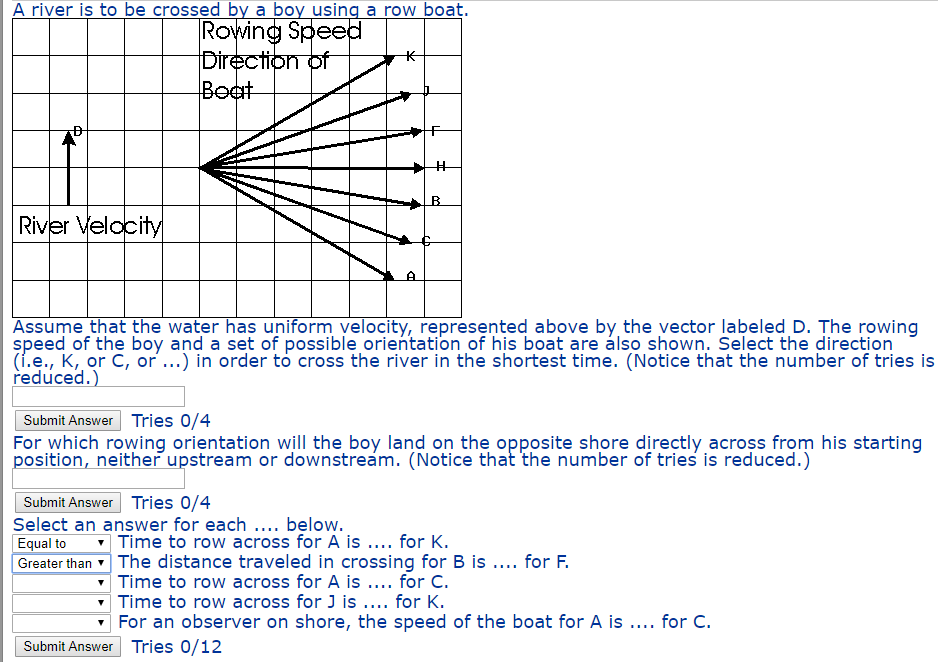Rowing Speed tion of River Velacity Assume that the water has uniform velocity, represented above by the vector labeled D. The rowing speed of the boy and a set of possible orientation of his boat are álso shown. Select the direction (ї.е., K,or C, or ) in order to cross the river in the shortest time. (Notice that the number of tries is reduced.) Submit Answer Tries 0/4 For which rowing orientation will the boy land on the opposite shore directly across from his starting position, neither upstream or downstreám. (Notice that the number of tries is reduced.) Submit Answer Tries 0/4 Select an answer for each.... below. Equal toTime to row across for A is for K. Greater than The distance traveled in crossing for B is.... for F Time to row across for A is for C. Time to row across forJ is... for K. For an observer on shore, the speed of the boat for A is.. for C Submit Answer Tries 0/12

Concept used - Aiming with the current sweeps her much farther downstream than aiming against the current

----------------------------------------------------------------------------

direction to cross the river in shortest time is H

--------------------------------------------------------------------------------

Boy will land on the opposite shore for direction C

-------------------------------------------------------------------------------------------

Time to row across for A is equal to for K

The distance traveled in crossing for B is less than for F

time to row across for A is more than for C

time to row across for J is less than K

For an observer on shore, the speed of boat for A is more than for C (not sure about this one)

##### Add Answer of: Rowing Speed tion of River Velacity Assume that the water has uniform velocity, represented above by the vector labeled...
Similar Homework Help Questions
• ### .Can't figure out the last set! Please help! :) Rowin River Vel㏄ Assume that the water...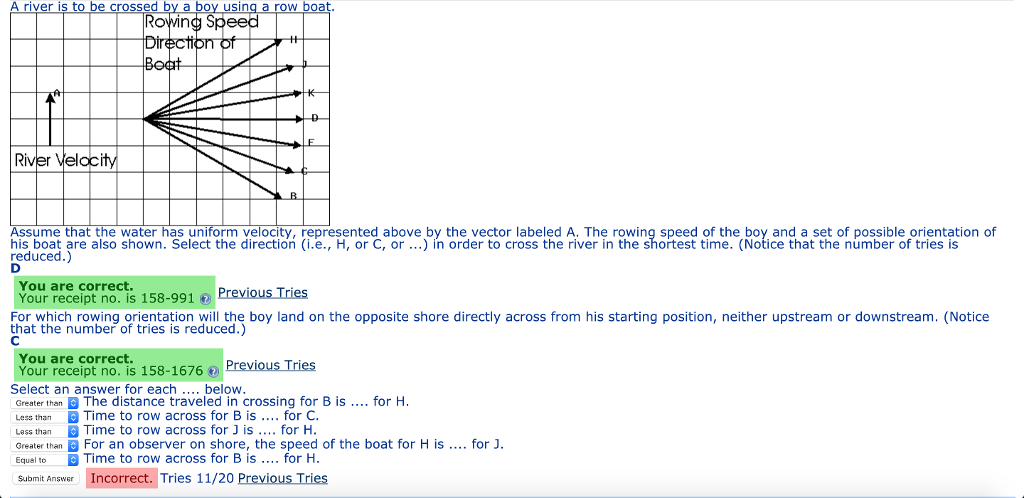.Can't figure out the last set! Please help! :) Rowin River Vel㏄ Assume that the water has uniform velocity, represented above by the vector labeled A. The rowing speed of the boy and a set of possible orientation of his boat are also shown. Select the direction (i.е., H, or C, or ) in order to cross the river in the shortest time. (Notice that the number of tries is reduced.) You are correct Your receipt no. is 158-991 For...

• ### Drection o Boat ver velocity, represented above by the vector labeled A. The rowing speed of...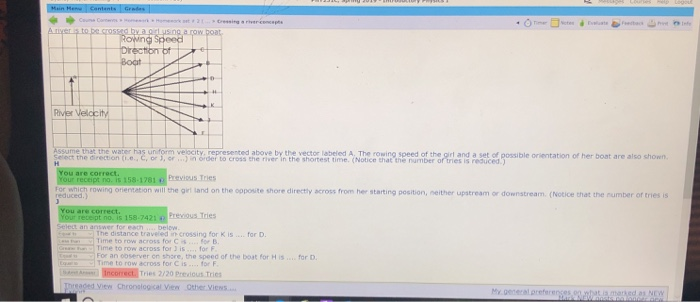Drection o Boat ver velocity, represented above by the vector labeled A. The rowing speed of the girl and a set of t the direct on (i e., С, or J, or …) n order to cross the river in the shortest time.(Notice thatthe namber or nes is reduced le orientation of her boat are also shown Previous Tries rowing orenteation will the girt land on the opposite shore directly scross from her starting position, neither upstream or downstream. (Notice...

• ### A river is to be crossed by a girl using a row boat. Rowing Spee Directipn...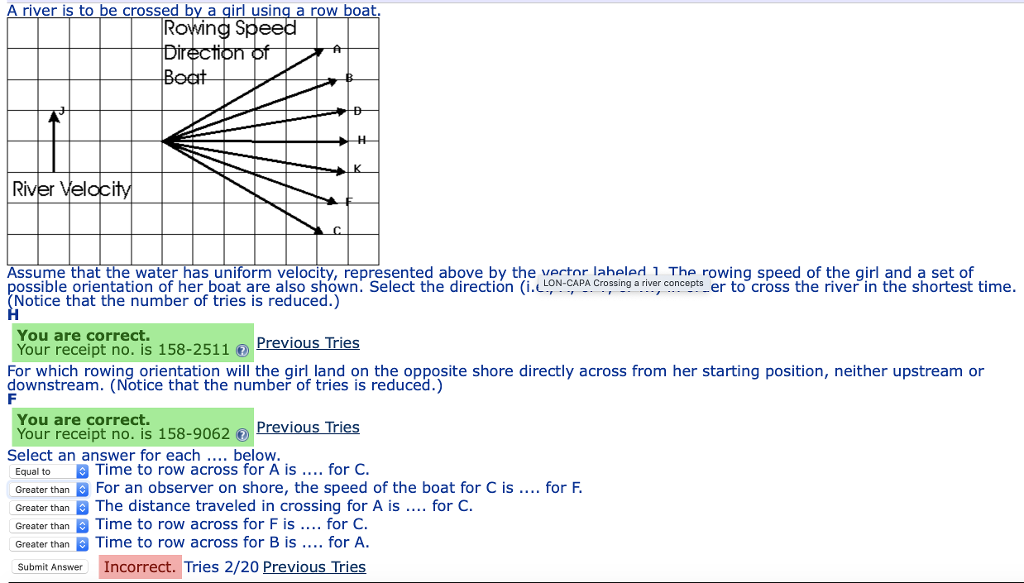A river is to be crossed by a girl using a row boat. Rowing Spee Directipn LK River Velocity Assume that the water has uniform velocity, represented above by the vector laheled The rowing speed of the girl and a set of ossible orientation of her boat are also shown. Select the direction (i사 4- Notice that the number of tries is reduced.) ACrossingar ver concepts er to cross the river in the shortest time. You are correct. Your receipt...

• ### A river is t crossed by a boy using a row boat Ri el Assume that...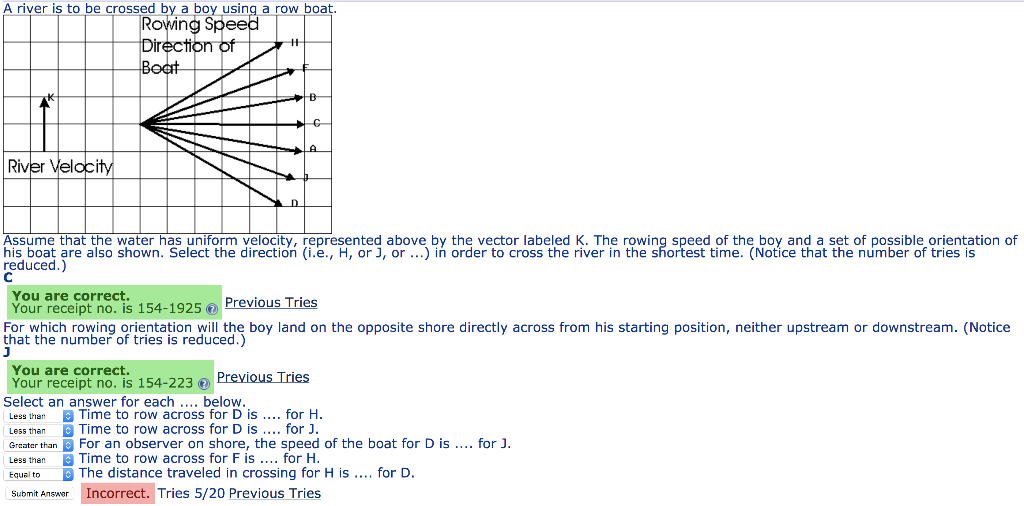A river is t crossed by a boy using a row boat Ri el Assume that the water has uniform velocity, represented above by the vector labeled K. The rowing speed of the boy and a set of possible orientation of his boat are also shown. Select the direction (i.e., H, or J, or ) in order to cross the river in the shortest time. (Notice that the number of tries is reduced.) You are correct. Your receipt no. is...

• ### A river is to be crossed by a girl using a row boat. Assume that the...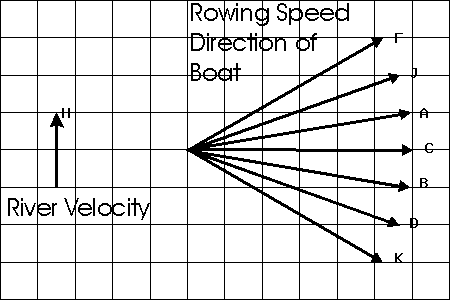A river is to be crossed by a girl using a row boat. Assume that the water has uniform velocity, represented above by the vector labeled H. The rowing speed of the girl and a set of possible orientation of her boat are also shown. Select the direction (i.e., F, or D, or ...) in order to cross the river in the shortest time. (Notice that the number of tries is reduced.) For which rowing orientation will the girl land...

• ### answer for part three are either (less than/equal to/greater than) Main Menu Contents Grades HW #2 (05/30 Th 11:59 P...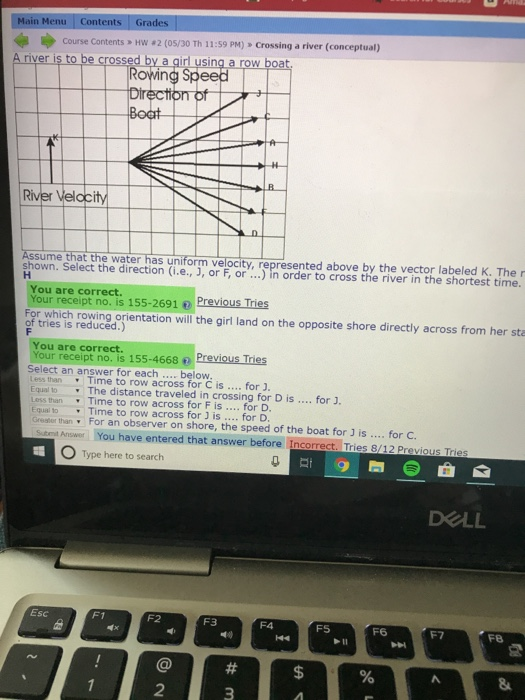answer for part three are either (less than/equal to/greater than) Main Menu Contents Grades HW #2 (05/30 Th 11:59 PM) 》 Crossing a river (conceptual) Course Contents Ariver is to be cross by a girl using a row River Assume that the water has uniform velocity, represented above by the vector labeled K. The r shown. Select the direction (i.e., J, or F, or in order to cross the river in the shortest time. You are correct. Your receipt no....

• ### VectorsA river is to be crossed by a girl using a row boat.Assume that the water has uniform velocity, represented above bythe vector labeled F. The rowing speed of the girl and a set ofpossible orientation of her boat arealso shown.Select the direction (i.e., J, or K, or ...) in order to cross theriver in the shortest time. (4 tries allowed.)

Need Online Homework Help?